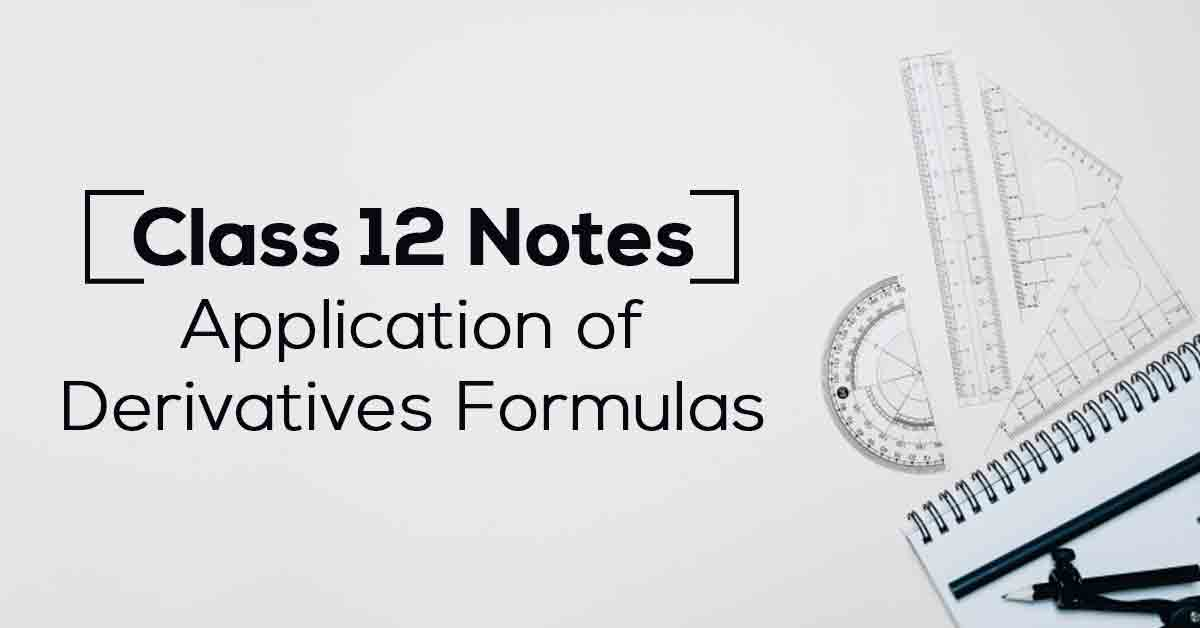Application of Derivatives Class 12 Notes | Vidyakul
×

# Application of Derivatives Class 12 Notes## Class 12 Maths Chapter 6 Application of Derivatives Formulas – PDF Download

In mathematical analysis, the maxima and minima (the respective plurals of maximum and minimum) of a function, known collectively as extrema (the plural of extremum), are the largest and smallest value of the function, either within a given range (the local or relative extrema) or on the entire domain of a function. Know More about these in Application of Derivatives Class 12 Notes List.

The topics and sub-topics covered in Application of Derivatives Class 12 Notes are:

6.1 Introduction

6.2 Rate of Change of Quantities

6.3 Increasing and Decreasing Functions

6.4 Tangents and Normals

6.5 Approximations

6.6 Maxima and Minima

6.6.1 Maximum and Minimum Values of a Function in a Closed Interval.

Download the FREE PDF of Application of Derivatives Class 12 Notes and start your preparation with Vidyakul!